第八节，配置分布式TensorFlow

由于随着神经网络层数的增多，需要训练的参数也会增多，随之而来需要的数据集就会很大，这样会造成需要更大的运算资源，而且还要消耗很长的运算时间。TensorFlow提供了一个可以分布式部署的模式，将一个训练任务拆分成多个小任务，配置到不同的计算机上完成协同运算，这样使用计算机群运算来代替单机运算，可以使训练时间大幅度缩短。

一 分布式TensorFlow角色以及原理

• ps：作为分布式训练的服务端，等到各个终端(supervisors)来连接。
• worker：在TensorFlow的代码注释中被称为supervisors，作为分布式训练的运算终端。
• chief supervisors：在众多运算终端中必须选中一个作为主要的运算终端。该终端是在运算终端中最先启动的，它的功能是合并各个终端运算后的学习参数，将其保存再写入。

• 服务器端作为一个多方协调者，等待各个运算终端来连接。
• cheif supervisors会在启动时统一管理全局的学习参数，进行初始化或从模型载入。
• 其它的运算终端只是负责得到其相应的任务并进行计算，并不会保存检查点以及用于TensorBoard可视化的summary日志等任何参数信息。

三 使用TensorFlow实现分布式部署训练

1.为每个角色创建IP地址和端口，创建server

'''
(1)为每个角色添加IP地址和端口，创建server
'''

'''定义IP和端口号'''
#指定服务器ip和port
strps_hosts = '127.0.0.1:1234'
#指定两个终端的ip和port
strworker_hosts =  '127.0.0.1:1235,127.0.0.1:1236'

#定义角色名称
strjob_name = 'ps'
#将字符串转为数组
ps_hosts = strps_hosts.split(',')
worker_hosts = strworker_hosts.split(',')

cluster_spec= tf.train.ClusterSpec({'ps':ps_hosts,'worker':worker_hosts})

#创建Server
server = tf.train.Server(
cluster_spec,
job_name = strjob_name,
task_index = task_index)

2.为ps角色添加等待函数

ps角色使用server.join()函数进行线程挂起，开始接受连接消息。

'''
(2) 为ps角色添加等待函数
'''
#ps角色处于监听状态，等待终端连接
if strjob_name == 'ps':
print('waiting....')
server.join()   

3.创建网络结构

'''
(3) 创建网络结构
'''
#设定训练集数据长度
n_train = 100

#生成x数据，[-1,1]之间，均分成n_train个数据
train_x = np.linspace(-1,1,n_train).reshape(n_train,1)

#把x乘以2，在加入(0,0.3)的高斯正太分布
train_y = 2*train_x + np.random.normal(loc=0.0,scale=0.3,size=[n_train,1])

#绘制x,y波形
plt.figure()
plt.plot(train_x,train_y,'ro',label='y=2x')   #o使用圆点标记一个点
plt.legend()
plt.show()

with tf.device(tf.train.replica_device_setter(
cluster = cluster_spec)):

'''
前向反馈
'''
#创建占位符
input_x = tf.placeholder(dtype=tf.float32)
input_y = tf.placeholder(dtype=tf.float32)

#模型参数
w = tf.Variable(tf.truncated_normal(shape=,mean=0.0,stddev=1),name='w')    #设置正太分布参数  初始化权重
b = tf.Variable(tf.truncated_normal(shape=,mean=0.0,stddev=1),name='b')    #设置正太分布参数  初始化偏置

#创建一个global_step变量
global_step = tf.train.get_or_create_global_step()

#前向结构
pred = tf.multiply(w,input_x) + b

#将预测值以直方图形式显示，给直方图命名为'pred'
tf.summary.histogram('pred',pred)

'''
反向传播bp
'''
#定义代价函数  选取二次代价函数
cost = tf.reduce_mean(tf.square(input_y - pred))

#将损失以标量形式显示 该变量命名为loss_function
tf.summary.scalar('loss_function',cost)

#设置求解器 采用梯度下降法 学习了设置为0.001 并把global_step变量放到优化器中，这样每运行一次优化器，globle_step就会自动获得当前迭代的次数

saver = tf.train.Saver(max_to_keep = 1)

#合并所有的summary
merged_summary_op = tf.summary.merge_all()

#初始化所有变量，因此变量需要放在其前面定义
init  =tf.global_variables_initializer()

4.创建Supervisor，管理session

'''
(4)创建Supervisor，管理session
'''
training_epochs = 2000
display_step = 20

sv = tf.train.Supervisor(is_chief = (task_index == 0),          #0号worker为chief
logdir='./LinearRegression/super/',    #检查点和summary文件保存的路径
init_op = init,  #初始化所有变量
summary_op = None,                            #summary_op用于自动保存summary文件，设置为None，表示不自动保存
saver = saver,   #将保存检查点的saver对象传入，supervisor会自动保存检查点文件。否则设置为None
global_step = global_step,
save_model_secs = 50   #保存检查点文件的时间间隔
)
• 在tf.train.Supervisor()函数中，is_cheif表明了是否为cheif supervisors角色，这里将task_index = 0的worker设置成chief supervisors。
• logdir：为检查点和summary日志文件的保存路径。不过这个似乎启动就会去这个logdir的目录去看有没有checkpoint的文件， 有的话就自动装载了，没有就用init_op指定的初始化参数。
• init_op：表示使用初始化变量的函数。
• summary_op:将保存summary的对象传入，就会自动保存summary文件。这里设置为None,表示不自动保存。
• saver：将保存检查点的saver对象传入，Supervisor就会自动保存检查点文件。如果不想自动保存，就设置为None。
• global_step：为迭代次数。
• save_model_op：为保存检查点文件的时间间隔，这里设置成50，表明每50秒自动保存一次检查点文件。为了使程序运行时间长一些，我们更改了training_epochs参数。

5.迭代训练

session中的内容和之前的一样，直接迭代训练即可，由于使用了Supervisor管理session，将使用sv.summary_computed函数来保存summary文件，同样，如果想要手动保存监测点文件，也可以使用sv.saver.save()函数。

'''
(5) 迭代训练
'''
#连接目标角色创建session
with sv.managed_session(server.target) as sess:
print("sess ok：")
print(global_step.eval(session=sess))
print('开始迭代：')

#存放批次值和代价值
plotdata = {'batch_size':[],'loss':[]}

#开始迭代 这里step表示当前执行步数，迭代training_epochs轮  需要执行training_epochs*n_train步
for step in range(training_epochs*n_train):
for (x,y) in zip(train_x,train_y):
#开始执行图  并返回当前步数
_,step = sess.run([train,global_step],feed_dict={input_x:x,input_y:y})

#生成summary
summary_str = sess.run(merged_summary_op,feed_dict={input_x:x,input_y:y})
#将summary写入文件  手动保存summary日志文件
sv.summary_computed(sess,summary_str,global_step = step)

#一轮训练完成后 打印输出信息
if step % display_step == 0:
#计算代价值
loss = sess.run(cost,feed_dict={input_x:train_x,input_y:train_y})
print('step {0}  cost {1}  w {2}  b{3}'.format(step,loss,sess.run(w),sess.run(b)))

#保存每display_step轮训练后的代价值以及当前迭代轮数
if not loss == np.nan:
plotdata['batch_size'].append(step)
plotdata['loss'].append(loss)

print('Finished!')
#手动保存检查点文件
#sv.saver.save(sess,'./LinearRegression/sv/sv.cpkt',global_step = step)

sv.stop()
• 在设置了自动保存检查点文件后，手动保存仍然有效。程序里我们在Supervisor对象创建的时候指定了自动保存检查点文件，程序里被我注释掉的最后一行是采用手动保存检查点文件。
• 在Supervisor对象创建的时候指定了不自动保存summary日志文件，我们采用了手动保存，调用了sv.summary_computed()函数。
• 在运行一半后终止，再运行Supervisor时会自动载入模型的参数，不需要手动调用saver.restore()。
• 在session中，不需要再运行tf.global_variables_initializer()函数。因为在Supervisor建立的时候回调用传入的init_op进行初始化，如果加了sess.run(tf.global_variables_initializer())，则会导致所载入模型的变量被二次清空。

6.建立worker文件

worker1.py文件修改如下：

#定义角色名称
strjob_name = 'worker'
task_index = 0

worker2.py文件修改如下：

#定义角色名称
strjob_name = 'worker'
task_index = 1

• worker2文件中不能使用sv.summary_computed()函数，因为worker2不是chief supervisors，在worker2中是不会为Supervisor对象构造默认summary_writer(所有的summary日志信息都要通过该对象进行写)对象的，所以即使程序调用sv.summary_computed()也无法执行下去，程序会报错。
• 手写控制summary日志和检查点文件保存时，需要将chief supervisors以外的worker全部去掉才可以，可以使用Supervisor按时间间隔保存的形式来管理，这样用一套代码就可以解决了。

7.部署运行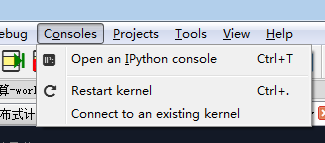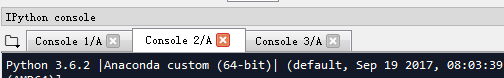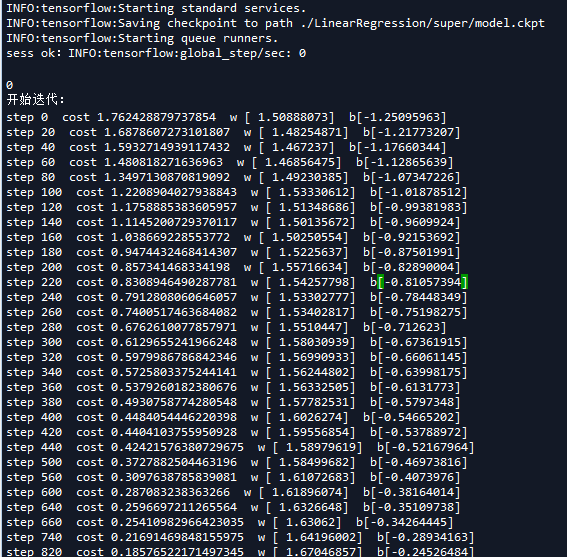worker2.py文件对应的窗口显示的信息如下：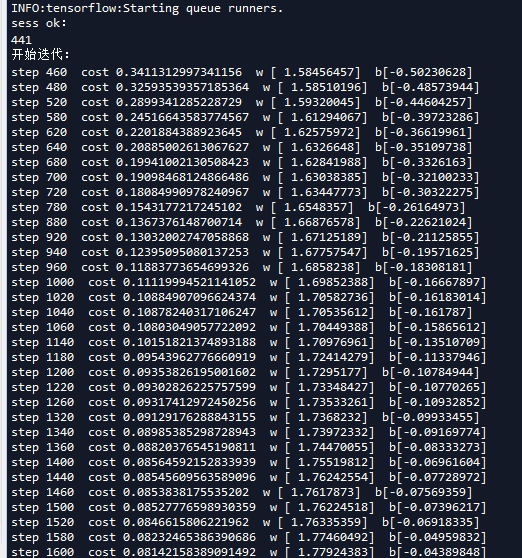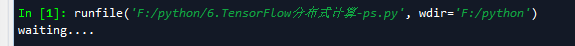四 最后再补充一些名词解释

• 客户端是一个用于建立TensorFlow计算图并创立与集群进行交互的会话层tensorflow::Session 的程序。一般客户端是通过python或C++实现的。一个独立的客户端进程可以同时与多个TensorFlow的服务端相连 ，同时一个独立的服务端也可以与多个客户端相连。

• 一个TensorFlow的集群里包含了一个或多个作业(job), 每一个作业又可以拆分成一个或多个任务(task)。集群的概念主要用与一个特定的高层次对象中，比如说训练神经网络，并行化操作多台机器等等。集群对象可以通过tf.train.ClusterSpec 来定义。

• 任务相当于是一个特定的TesnsorFlow服务端，其相当于一个独立的进程，该进程属于特定的作业并在作业中拥有对应的序号。
TensorFlow服务端(TensorFlow server) 。

ps.py完整代码：# -*- coding: utf-8 -*-
"""
Created on Thu Apr 19 08:52:30 2018

@author: zy
"""

import tensorflow as tf
import numpy as np
import os
import matplotlib.pyplot as plt

'''

'''

'''
(1)为每个角色添加IP地址和端口，创建server
'''

'''定义IP和端口号'''
#指定服务器ip和port
strps_hosts = '127.0.0.1:1234'
#指定两个终端的ip和port
strworker_hosts =  '127.0.0.1:1235,127.0.0.1:1236'

#定义角色名称
strjob_name = 'ps'
#将字符串转为数组
ps_hosts = strps_hosts.split(',')
worker_hosts = strworker_hosts.split(',')
cluster_spec = tf.train.ClusterSpec({'ps': ps_hosts,'worker': worker_hosts})

#创建server
server = tf.train.Server(
cluster_spec,
job_name = strjob_name,

'''
(2) 为ps角色添加等待函数
'''
#ps角色处于监听状态，等待终端连接
if strjob_name == 'ps':
print('waiting....')
server.join()

'''
(3) 创建网络结构
'''

#设定训练集数据长度
n_train = 100

#生成x数据，[-1,1]之间，均分成n_train个数据
train_x = np.linspace(-1,1,n_train).reshape(n_train,1)

#把x乘以2，在加入(0,0.3)的高斯正太分布
train_y = 2*train_x + np.random.normal(loc=0.0,scale=0.3,size=[n_train,1])

#绘制x,y波形
plt.figure()
plt.plot(train_x,train_y,'ro',label='y=2x')   #o使用圆点标记一个点
plt.legend()
plt.show()

#创建网络结构时，通过tf.device()函数将全部的节点都放在当前任务下
with tf.device(tf.train.replica_device_setter(
cluster = cluster_spec)):

'''
前向反馈
'''
#创建占位符
input_x = tf.placeholder(dtype=tf.float32)
input_y = tf.placeholder(dtype=tf.float32)

#模型参数
w = tf.Variable(tf.truncated_normal(shape=,mean=0.0,stddev=1),name='w')    #设置正太分布参数  初始化权重
b = tf.Variable(tf.truncated_normal(shape=,mean=0.0,stddev=1),name='b')    #设置正太分布参数  初始化偏置

#创建一个global_step变量
global_step = tf.train.get_or_create_global_step()

#前向结构
pred = tf.multiply(w,input_x) + b

#将预测值以直方图形式显示，给直方图命名为'pred'
tf.summary.histogram('pred',pred)

'''
反向传播bp
'''
#定义代价函数  选取二次代价函数
cost = tf.reduce_mean(tf.square(input_y - pred))

#将损失以标量形式显示 该变量命名为loss_function
tf.summary.scalar('loss_function',cost)

#设置求解器 采用梯度下降法 学习了设置为0.001 并把global_step变量放到优化器中，这样每运行一次优化器，global_step就会自动获得当前迭代的次数

saver = tf.train.Saver(max_to_keep = 1)

#合并所有的summary
merged_summary_op = tf.summary.merge_all()

#初始化所有变量，因此变量需要放在其前面定义
init  =tf.global_variables_initializer()

'''
(4)创建Supervisor，管理session
'''
training_epochs = 2000
display_step = 20

sv = tf.train.Supervisor(is_chief = (task_index == 0),          #0号worker为chief
logdir='./LinearRegression/super/',    #检查点和summary文件保存的路径
init_op = init,  #初始化所有变量
summary_op = None,                            #summary_op用于自动保存summary文件，设置为None，表示不自动保存
saver = saver,   #将保存检查点的saver对象传入，supervisor会自动保存检查点文件。否则设置为None
global_step = global_step,
save_model_secs = 50   #保存检查点文件的时间间隔
)

'''
(5) 迭代训练
'''
#连接目标角色创建session
with sv.managed_session(server.target) as sess:
print("sess ok：")
print(global_step.eval(session=sess))
print('开始迭代：')

#存放批次值和代价值
plotdata = {'batch_size':[],'loss':[]}

#开始迭代 这里step表示当前执行步数，迭代training_epochs轮  需要执行training_epochs*n_train步
for step in range(training_epochs*n_train):
for (x,y) in zip(train_x,train_y):
#开始执行图  并返回当前步数
_,step = sess.run([train,global_step],feed_dict={input_x:x,input_y:y})

#生成summary
summary_str = sess.run(merged_summary_op,feed_dict={input_x:x,input_y:y})
#将summary写入文件  手动保存summary日志文件
sv.summary_computed(sess,summary_str,global_step = step)

#一轮训练完成后 打印输出信息
if step % display_step == 0:
#计算代价值
loss = sess.run(cost,feed_dict={input_x:train_x,input_y:train_y})
print('step {0}  cost {1}  w {2}  b{3}'.format(step,loss,sess.run(w),sess.run(b)))

#保存每display_step轮训练后的代价值以及当前迭代轮数
if not loss == np.nan:
plotdata['batch_size'].append(step)
plotdata['loss'].append(loss)

print('Finished!')
#手动保存检查点文件
#sv.saver.save(sess,'./LinearRegression/sv/sv.cpkt',global_step = step)

sv.stop()


View Code

worker1.py完整代码：# -*- coding: utf-8 -*-
"""
Created on Thu Apr 19 08:52:30 2018

@author: zy
"""

import tensorflow as tf
import numpy as np
import os
import matplotlib.pyplot as plt

'''

'''

'''
(1)为每个角色添加IP地址和端口，创建worker
'''

'''定义IP和端口号'''
#指定服务器ip和port
strps_hosts = '127.0.0.1:1234'
#指定两个终端的ip和port
strworker_hosts =  '127.0.0.1:1235,127.0.0.1:1236'

#定义角色名称
strjob_name = 'worker'
#将字符串转为数组
ps_hosts = strps_hosts.split(',')
worker_hosts = strworker_hosts.split(',')
cluster_spec = tf.train.ClusterSpec({'ps': ps_hosts,'worker': worker_hosts})

#创建server
server = tf.train.Server(
cluster_spec,
job_name = strjob_name,

'''
(2) 为ps角色添加等待函数
'''
#ps角色处于监听状态，等待终端连接
if strjob_name == 'ps':
print('waiting....')
server.join()

'''
(3) 创建网络结构
'''

#设定训练集数据长度
n_train = 100

#生成x数据，[-1,1]之间，均分成n_train个数据
train_x = np.linspace(-1,1,n_train).reshape(n_train,1)

#把x乘以2，在加入(0,0.3)的高斯正太分布
train_y = 2*train_x + np.random.normal(loc=0.0,scale=0.3,size=[n_train,1])

#绘制x,y波形
plt.figure()
plt.plot(train_x,train_y,'ro',label='y=2x')   #o使用圆点标记一个点
plt.legend()
plt.show()

#创建网络结构时，通过tf.device()函数将全部的节点都放在当前任务下
with tf.device(tf.train.replica_device_setter(
cluster = cluster_spec)):

'''
前向反馈
'''
#创建占位符
input_x = tf.placeholder(dtype=tf.float32)
input_y = tf.placeholder(dtype=tf.float32)

#模型参数
w = tf.Variable(tf.truncated_normal(shape=,mean=0.0,stddev=1),name='w')    #设置正太分布参数  初始化权重
b = tf.Variable(tf.truncated_normal(shape=,mean=0.0,stddev=1),name='b')    #设置正太分布参数  初始化偏置

#创建一个global_step变量
global_step = tf.train.get_or_create_global_step()

#前向结构
pred = tf.multiply(w,input_x) + b

#将预测值以直方图形式显示，给直方图命名为'pred'
tf.summary.histogram('pred',pred)

'''
反向传播bp
'''
#定义代价函数  选取二次代价函数
cost = tf.reduce_mean(tf.square(input_y - pred))

#将损失以标量形式显示 该变量命名为loss_function
tf.summary.scalar('loss_function',cost)

#设置求解器 采用梯度下降法 学习了设置为0.001 并把global_step变量放到优化器中，这样每运行一次优化器，global_step就会自动获得当前迭代的次数

saver = tf.train.Saver(max_to_keep = 1)

#合并所有的summary
merged_summary_op = tf.summary.merge_all()

#初始化所有变量，因此变量需要放在其前面定义
init  =tf.global_variables_initializer()

'''
(4)创建Supervisor，管理session
'''
training_epochs = 2000
display_step = 20

sv = tf.train.Supervisor(is_chief = (task_index == 0),          #0号worker为chief
logdir='./LinearRegression/super/',    #检查点和summary文件保存的路径
init_op = init,  #初始化所有变量
summary_op = None,                            #summary_op用于自动保存summary文件，设置为None，表示不自动保存
saver = saver,   #将保存检查点的saver对象传入，supervisor会自动保存检查点文件。否则设置为None
global_step = global_step,
save_model_secs = 50   #保存检查点文件的时间间隔
)

'''
(5) 迭代训练
'''
#连接目标角色创建session
with sv.managed_session(server.target) as sess:
print("sess ok：")
print(global_step.eval(session=sess))
print('开始迭代：')

#存放批次值和代价值
plotdata = {'batch_size':[],'loss':[]}

#开始迭代 这里step表示当前执行步数，迭代training_epochs轮  需要执行training_epochs*n_train步
for step in range(training_epochs*n_train):
for (x,y) in zip(train_x,train_y):
#开始执行图  并返回当前步数
_,step = sess.run([train,global_step],feed_dict={input_x:x,input_y:y})

#生成summary
summary_str = sess.run(merged_summary_op,feed_dict={input_x:x,input_y:y})
#将summary写入文件  手动保存summary日志文件
sv.summary_computed(sess,summary_str,global_step = step)

#一轮训练完成后 打印输出信息
if step % display_step == 0:
#计算代价值
loss = sess.run(cost,feed_dict={input_x:train_x,input_y:train_y})
print('step {0}  cost {1}  w {2}  b{3}'.format(step,loss,sess.run(w),sess.run(b)))

#保存每display_step轮训练后的代价值以及当前迭代轮数
if not loss == np.nan:
plotdata['batch_size'].append(step)
plotdata['loss'].append(loss)

print('Finished!')
#手动保存检查点文件
#sv.saver.save(sess,'./LinearRegression/sv/sv.cpkt',global_step = step)

sv.stop()


View Code

worker2.py完整代码：# -*- coding: utf-8 -*-
"""
Created on Thu Apr 19 08:52:30 2018

@author: zy
"""

import tensorflow as tf
import numpy as np
import os
import matplotlib.pyplot as plt

'''

'''

'''
(1)为每个角色添加IP地址和端口，创建worker
'''

'''定义IP和端口号'''
#指定服务器ip和port
strps_hosts = '127.0.0.1:1234'
#指定两个终端的ip和port
strworker_hosts =  '127.0.0.1:1235,127.0.0.1:1236'

#定义角色名称
strjob_name = 'worker'
#将字符串转为数组
ps_hosts = strps_hosts.split(',')
worker_hosts = strworker_hosts.split(',')
cluster_spec = tf.train.ClusterSpec({'ps': ps_hosts,'worker': worker_hosts})

#创建server
server = tf.train.Server(
cluster_spec,
job_name = strjob_name,

'''
(2) 为ps角色添加等待函数
'''
#ps角色处于监听状态，等待终端连接
if strjob_name == 'ps':
print('waiting....')
server.join()

'''
(3) 创建网络结构
'''

#设定训练集数据长度
n_train = 100

#生成x数据，[-1,1]之间，均分成n_train个数据
train_x = np.linspace(-1,1,n_train).reshape(n_train,1)

#把x乘以2，在加入(0,0.3)的高斯正太分布
train_y = 2*train_x + np.random.normal(loc=0.0,scale=0.3,size=[n_train,1])

#绘制x,y波形
plt.figure()
plt.plot(train_x,train_y,'ro',label='y=2x')   #o使用圆点标记一个点
plt.legend()
plt.show()

#创建网络结构时，通过tf.device()函数将全部的节点都放在当前任务下
with tf.device(tf.train.replica_device_setter(
cluster = cluster_spec)):

'''
前向反馈
'''
#创建占位符
input_x = tf.placeholder(dtype=tf.float32)
input_y = tf.placeholder(dtype=tf.float32)

#模型参数
w = tf.Variable(tf.truncated_normal(shape=,mean=0.0,stddev=1),name='w')    #设置正太分布参数  初始化权重
b = tf.Variable(tf.truncated_normal(shape=,mean=0.0,stddev=1),name='b')    #设置正太分布参数  初始化偏置

#创建一个global_step变量
global_step = tf.train.get_or_create_global_step()

#前向结构
pred = tf.multiply(w,input_x) + b

#将预测值以直方图形式显示，给直方图命名为'pred'
tf.summary.histogram('pred',pred)

'''
反向传播bp
'''
#定义代价函数  选取二次代价函数
cost = tf.reduce_mean(tf.square(input_y - pred))

#将损失以标量形式显示 该变量命名为loss_function
tf.summary.scalar('loss_function',cost)

#设置求解器 采用梯度下降法 学习了设置为0.001 并把global_step变量放到优化器中，这样每运行一次优化器，global_step就会自动获得当前迭代的次数

saver = tf.train.Saver(max_to_keep = 1)

#合并所有的summary
merged_summary_op = tf.summary.merge_all()

#初始化所有变量，因此变量需要放在其前面定义
init  =tf.global_variables_initializer()

'''
(4)创建Supervisor，管理session
'''
training_epochs = 2000
display_step = 20

sv = tf.train.Supervisor(is_chief = (task_index == 0),          #0号worker为chief
logdir='./LinearRegression/super/',    #检查点和summary文件保存的路径
init_op = init,  #初始化所有变量
summary_op = None,                            #summary_op用于自动保存summary文件，设置为None，表示不自动保存
saver = saver,   #将保存检查点的saver对象传入，supervisor会自动保存检查点文件。否则设置为None
global_step = global_step,
save_model_secs = 50   #保存检查点文件的时间间隔
)

'''
(5) 迭代训练
'''
#连接目标角色创建session
with sv.managed_session(server.target) as sess:
print("sess ok：")
print(global_step.eval(session=sess))
print('开始迭代：')

#存放批次值和代价值
plotdata = {'batch_size':[],'loss':[]}

#开始迭代 这里step表示当前执行步数，迭代training_epochs轮  需要执行training_epochs*n_train步
for step in range(training_epochs*n_train):
for (x,y) in zip(train_x,train_y):
#开始执行图  并返回当前步数
_,step = sess.run([train,global_step],feed_dict={input_x:x,input_y:y})

#生成summary
summary_str = sess.run(merged_summary_op,feed_dict={input_x:x,input_y:y})
#将summary写入文件  手动保存summary日志文件
#sv.summary_computed(sess,summary_str,global_step = step)

#一轮训练完成后 打印输出信息
if step % display_step == 0:
#计算代价值
loss = sess.run(cost,feed_dict={input_x:train_x,input_y:train_y})
print('step {0}  cost {1}  w {2}  b{3}'.format(step,loss,sess.run(w),sess.run(b)))

#保存每display_step轮训练后的代价值以及当前迭代轮数
if not loss == np.nan:
plotdata['batch_size'].append(step)
plotdata['loss'].append(loss)

print('Finished!')
#手动保存检查点文件
#sv.saver.save(sess,'./LinearRegression/sv/sv.cpkt',global_step = step)

sv.stop()
View Code
posted @ 2018-04-19 14:25 大奥特曼打小怪兽 阅读(...) 评论(...) 编辑 收藏